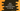# Java program to print random uppercase letter in a string### Java program to print random uppercase letter in a String :

In this tutorial, we will learn how to print random uppercase letter from a String in Java. To achieve that, we will first create one random number . The size of the random number is maximum length of the string. After that, we will pick the character for that position from the String and finally, we will print the uppercase version of the character. The java program is as below :

### Java program to print random Uppercase character :

``````import java.util.*;

public class Main {

public static void main(String[] args) {
//1
String myString = "HelloWorld";

//2
Random randomNumber = new Random();

//3
for (int i = 0; i < 10; i++) {
//4
int randomNo = randomNumber.nextInt(myString.length());

//5
Character character = myString.charAt(randomNo);

//6
System.out.println("Random Character : " + Character.toUpperCase(character));
}
}

}``````

### Explanation :

_ The commented numbers in the above program denotes the step number below : _

1. String is given and stored in the variable myString.
2. Create one Random object to create random number.
3. Run one for loop to run for 10 times. We will print one random character each time.
4. Create one random number using the Random object created on step - 2. The object will create maximum number 7 for this example since size of string myString is 8.
5. Get the character from the string for that random position we have calculated in the above step.
6. Print out the uppercase character by converting the character to upper case

### Output :

``````Random Character : E
Random Character : R
Random Character : R
Random Character : O
Random Character : E
Random Character : D
Random Character : L
Random Character : O
Random Character : D
Random Character : D``````

The output will different for your case, because it will pick random character for each of these 10 steps.